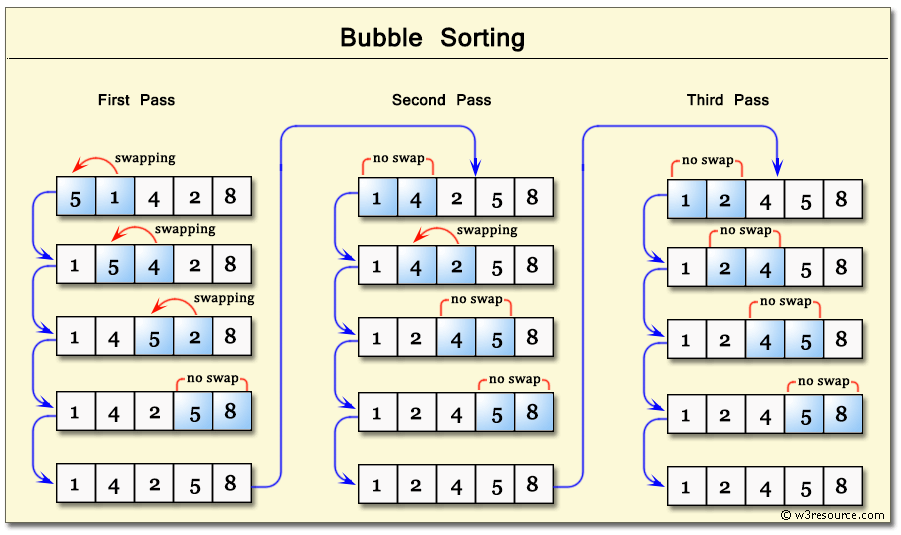# Sorting Algorithm - Bubble sort Implementation

Sorting is a famous problem in programming, today I will share the way I have implemented the Bubble Sort Algorithm.

## Bubble Sort

Bubble Sort is the algorithm to sort elements in an array by ascending or descending. The algorithm will partition the array into a sorted partition and unsorted partition.## Implementation

The algorithm is very simple, we repeatedly swap the adjacent elements if they are in the wrong order.

#### Ascending

• Init a variable called `lastUnsortedIndex`. Its value is the last index of an unsorted array - which is `arr.length - 1`.

• We are going to compare two values of the current index and the next index, so we will need two loops. The first loop will start increasing from 0 to `lastUnsortedIndex`, the second loop will start increasing from 0 to the index before `lastUnsortedIndex` which is `lastUnsortedIndex - i` (i is the index from the first loop). Swap the values if the current index greater than the next index.

• The algorithm ends when i == lastUnsortedIndex

• The sorted array direction is ascending

Implementation